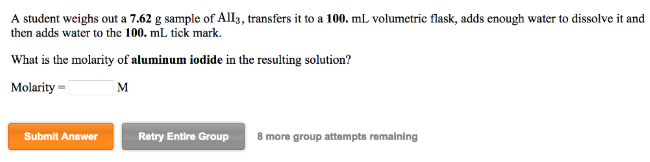# Problem: A student weighs out a 7.62 g sample of AlI3, transfers it to a 100. mL volumetric flask, adds enough water to dissolve it and then adds water to the 100. mL tick mark. What is the molarity of aluminum iodide in the resulting solution? Molarity = _____ M

###### FREE Expert Solution
99% (334 ratings)###### Problem Details

A student weighs out a 7.62 g sample of AlI3, transfers it to a 100. mL volumetric flask, adds enough water to dissolve it and then adds water to the 100. mL tick mark. What is the molarity of aluminum iodide in the resulting solution?

Molarity = _____ M